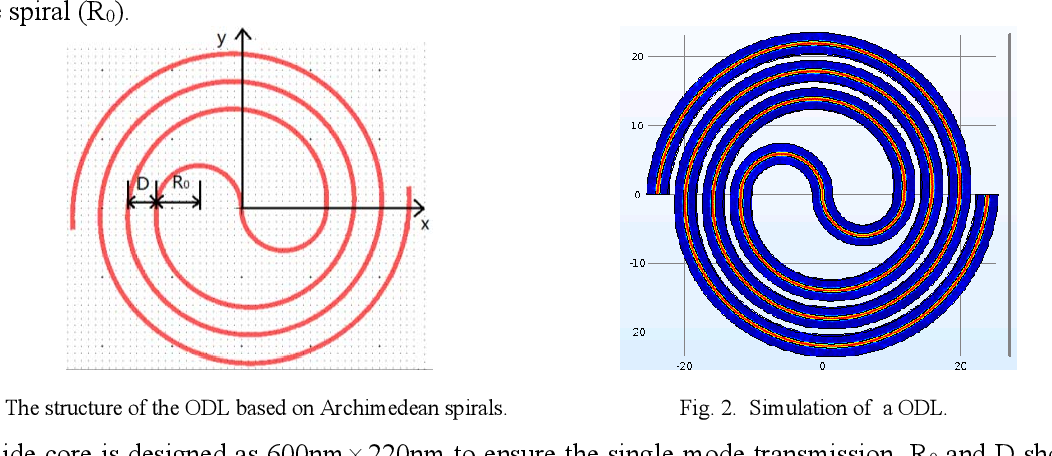## Photonics

Topics related to Lumerical and more

•Nathan Lin
Subscriber

Ultralow-loss compact silicon photonic waveguide spirals and delay lines (optica.org)

I saw a structure like this and would like to simulate this structure on FDTD, what is the best suggested approach for the simulation setup, how to draw this on Lumerical, and the recommended solver?•Amrita Pati
Ansys Employee

Hi Nathan,

The simplest approach would be to use the polygon object. If you have the equation of the spiral then you can set the vertices of the polygon using the equation. It is important to note that linear interpolation is used between the neighbouring vertices of the polygon. But if you have sufficiently large number of points/vertices then the structure should be smooth enough.

Regards,
Amrita

•Nathan Lin
Subscriber

Thanks, as for the SIM, since the line enters from the outer-most loop and exits the outer-most loop as well (see picture below), do we need FDTD for such SIM?•Amrita Pati
Ansys Employee

Hi Nathan,

You can use FDTD to calculate different results such as the transmission, losses etc and how they change with different physical parameters of the structure. If the structure is mostly planar, and there is minimal vertical coupling of modes then you can use varFDTD, which reduces a 3D simulation to effectively a 2D one, thereby also reducing the memory/time requirements. If you want to perform circuit level simulation, then you can use INTERCONNECT.

Regards,
Amrita